Next: The Trifocal Tensor Up: Linear Approaches Previous: Algebraic Projective Geometry

## Epipolar Geometry

The application of projective geometry techniques in computer vision is most notable in the Stereo Vision problem which is very closely related to Structure-from-Motion. Unlike general motion, stereo vision assumes that there are only two shots of the scene. In principle, then, one could apply stereo vision algorithms to a structure from motion task.

Applying projective geometry to stereo vision is not new and can be traced back from 19th century photogrammetry to work in the late sixties by Thompson . However, interest in the subject was recently rekindled in the computer vision community thanks to important works in projective invariants and reconstruction by Faugeras  and Hartley .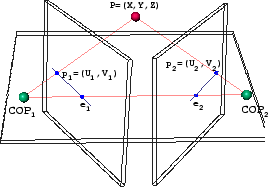Figure 4 depicts the imaging situation for stereo vision. The application of projective geometry to this situation results in the now popular epipolar geometry approach. The three points [COP1,COP2,P] form what is called an epipolar plane and the intersections of this plane with the two image planes form the epipolar lines. The line connecting the two centers of projection [COP1,COP2] intersects the image planes at the conjugate points e1 and e2 which are called epipoles. Assume that the 3D point P projects into the two image planes as the points p1 and p2 which are expressed in homogeneous coordinates (u1,v1,1) and (u2,v2,1) respectively. After some manipulations, the main result of the epipolar geometry is that the following linear relationship (Equation 3) can be written.

 p1t F p2 = 0 (3)

Here, F is the so-called fundamental matrix which is a 3 x 3 entity with 9 parameters. However, it is constrained to have rank 2 (i.e.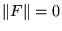) and can undergo an arbitrary scale factor. Thus, there are only 7 degrees of freedom in F. It defines the geometry of the correspondences between two views in a compact way, encoding intrinsic camera geometry as well as the extrinsic relative motion between the two cameras. Due to the linearity of the above equation, the epipolar geometry approach maintains a clean elegance in its manipulations. In addition, the structure of the scene is eliminated from the estimation of F and can be recovered in a separate step. Given the matrix F, identifying a point in one image identifies a corresponding epipolar line in the other image. 3

Hartley proposes an elegant technique for recovering the parameters of the fundamental matrix when at least 8 points are observed . Expanding the expression in Equation 3 gives one linear constraint on F per observed point as in Equation 4. Combining N of these equations from N corresponded features results in the linear system of the form A f = 0.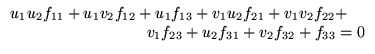(4)

Typically, one solves such a linear system using more than 8 points in a least squares minimization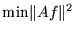subject to the constraint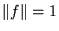. This constraint fixes the scale of the fundamental which otherwise is arbitrary. In addition, the rank 2 constraint must also be enforced. The algorithm employed utilizes an SVD computation but can be quite unstable. One way to alleviate this numerical ill-conditioning is to normalize pixel coordinates to span [-1,1]. For robust fundamental matrix estimation techniques, refer to .

The fundamental matrix F is recovered independently of the structure and can be useful on its own, for example in a robotics application . Hartley also uses it to derive Kruppa equations for recovering camera internal parameters  . Ultimately, it becomes possible to recover Euclidean 3D coordinates for the structure which are often desirable for most typical application purposes.

At this point it is worthwhile to study the stability of such techniques. The reader should consider the case where the centers of projection of both images are close to each other (COP1 and COP2). Note the degeneracy when the centers overlap, which is the case when there is no translation and only rotation. A point in one image does not project to an epipolar line in the other for these cases. Degeneracy also occurs when all 3D points in the scene are coplanar. The result is that it is not possible to determine the epipolar geometry between close consecutive frames and it cannot be determined from image correspondences alone. The linearization in epipolar geometry creates these degeneracies and numerical ill-conditioning near them. Therefore, one requires a large base-line or translation between the image planes for small errors. One way to overcome these degeneracies, is provided by Torr et al. . Their technique involves switching from epipolar feature matching to a homography approach which can automatically detect and handle degenerate cases such as pure camera rotation.

The linear epipolar geometry formulation also exhibits sensitivity to noise (i.e. in the 2D image measurements) when compared to nonlinear modeling approaches. One reason is that each point can be corresponded to any point along the epipolar line in the other image. Thus, the noise properties in the image are not isotropic with noise along the epipolar line remaining completely unpenalized. Thus, solutions tend to produce high residual errors along the epipolar lines and poor reconstruction. Experimental verification of this can be found in .Next: The Trifocal Tensor Up: Linear Approaches Previous: Algebraic Projective Geometry

1999-05-17